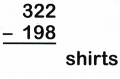Practice questions available in McGraw Hill Math Grade 2 Answer Key PDF Chapter 5 Test will engage students and is a great way of informal assessment.

Write the sum

Question 1.
28 + 34 = ____________
34 + 28 = ____________
28 + 34 = 62
34 + 28 = 62

Explanation:
The order of the addends may differ but the sum will remain same.

Question 2.
58 + 13 = ____________
13 + 58 = ____________
58 + 13 = 71
13 + 58 = 71

Explanation:
The order of the addends may differ but the sum will remain same.

Question 3.
44 + 23 + 11 = ____________
23 + 11 + 44 = ____________
44 + 23 + 11 = 78
23 + 11 + 44 = 78

Explanation:
The order of the addends may differ but the sum will remain same.

Question 4.
228 + 37 + 9 = ____________
37 + 9 + 228 = ____________
228 + 37 + 9 = 274
37 + 9 + 228 = 274

Explanation:
The order of the addends may differ but the sum will remain same.

Question 5.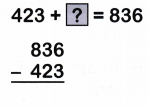423 + __________ = 836
423 + 413 = 836

Explanation:
423 + ? = 836
Subtract the addend from the sum
836 – 423 = ?
The difference is the missing addend
836 – 423 = 413
So, the missing addend is 413
Therefore, 836 + 423 = 413

Question 6.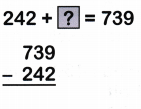242 + ___________ = 739
242 + 497 = 739

Explanation:
242 + ? = 739
Subtract the addend from the sum
739 – 242 = ?
The difference is the missing addend
739 – 242 = 497
So, the missing addend is 497
Therefore, 242 + 497 = 739.

Question 7.671 + __________ = 948
671 + 277 = 948

Explanation:
671 + ? = 948
Subtract the addend from the sum
948 – 671 = ?
The difference is the missing addend
948 – 671 = 277
So, the missing addend is 277
Therefore, 671 + 277 = 948.

Question 8.396 + __________ = 600
396 + 204 = 600

Explanation:
396 + ? = 600
Subtract the addend from the sum
600 – 396 = ?
The difference is the missing addend
600 – 396 = 204
So, the missing addend is 204
Therefore, 396 + 204 = 600.

Question 9.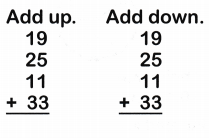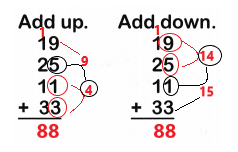Explanation:
We group numbers as we add
The sum of 19, 25, 11 and 33 is 88.

Question 10.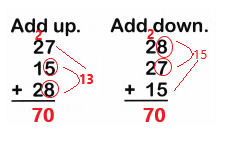Explanation:
We group numbers as we add
The sum of 27, 15 and 28 is 70.

Use mental math to add or subtract. Write the sum or difference.

Question 11.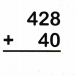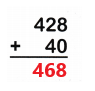Explanation:
Use mental math and add only tens
20 + 40 = 60
The sum of 428 and 40 is 468

Question 12.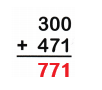Explanation:
Use mental math and add only hundreds
300 + 400 = 700
So, the sum of 300 and 471 is 771.

Question 13.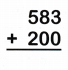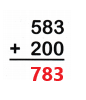Explanation:
Use mental math and add only hundreds
500 + 200 = 700
So, the sum of 583 and 200 is 783.

Question 14.Explanation:
Use mental math and subtract only tens
80 – 30 = 50
So, the difference of 483 and 30 is 453.

Question 15.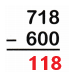Explanation:
Use mental math and subtract only hundreds
700 – 600 = 100
So, the difference of 718 and 600 is 118.

Question 16.Explanation:
Use mental math and subtract only hundreds
700 – 100 = 600
So, he difference of 700 and 100 is 600.

Solve

Question 17.
A store had 322 shirts. 198 shirts were sold. How many shirts are left in the store?